Algebra 1 3-5 Complete Lesson: Working With Sets
starstarstarstarstarstarstarstarstarstar
5 (1 rating)
by Matthew Richardson
| 29 Questions
Note from the author:
A complete formative lesson with embedded slideshow, mini lecture screencasts, checks for understanding, practice items, mixed review, and reflection. I create these assignments to supplement each lesson of Pearson's Common Core Edition Algebra 1, Algebra 2, and Geometry courses. See also mathquest.net and twitter.com/mathquestEDU.The outlined content above was added from outside of Formative.1
1
10 pts
Solve It! Most numbers on a telephone keypad correspond to a set of letters. Suppose you're friend is using the keypad to encrypt a secret message. He sends you the string of characters 4 6 6 3 in that order. What is one word he might be trying to send?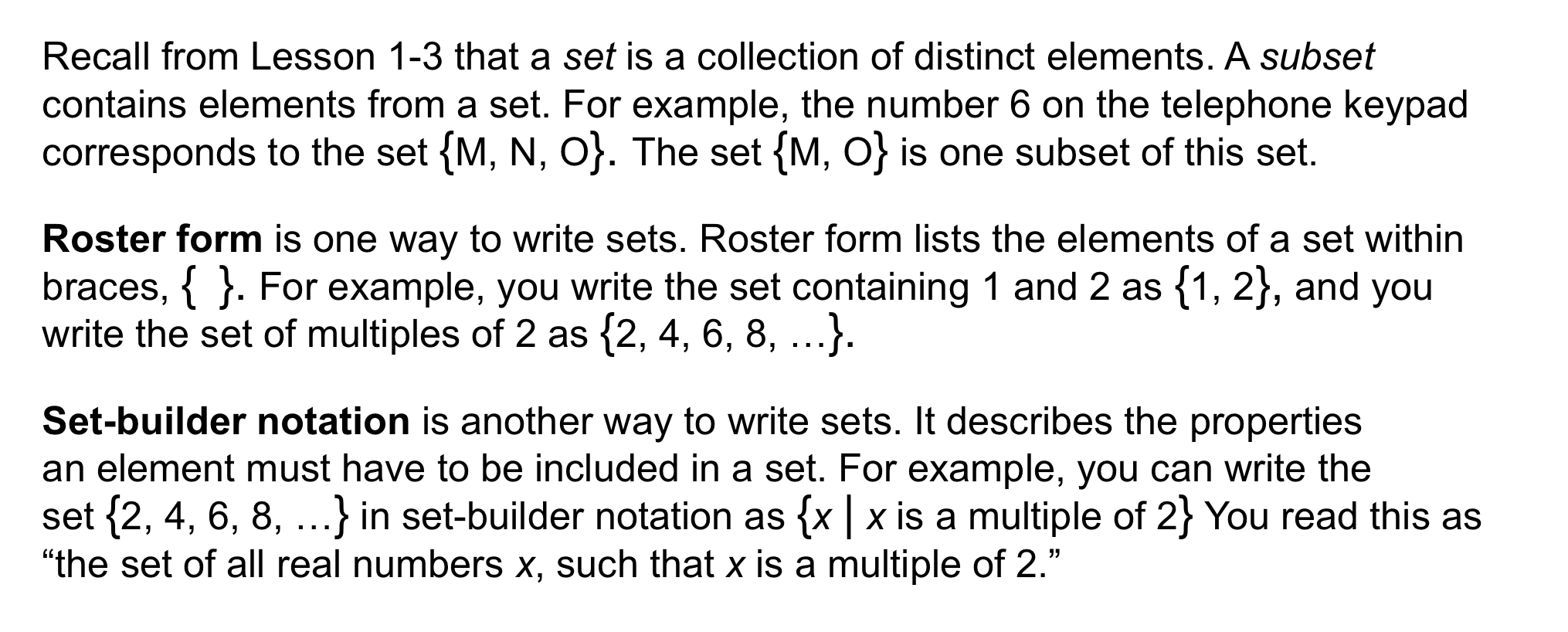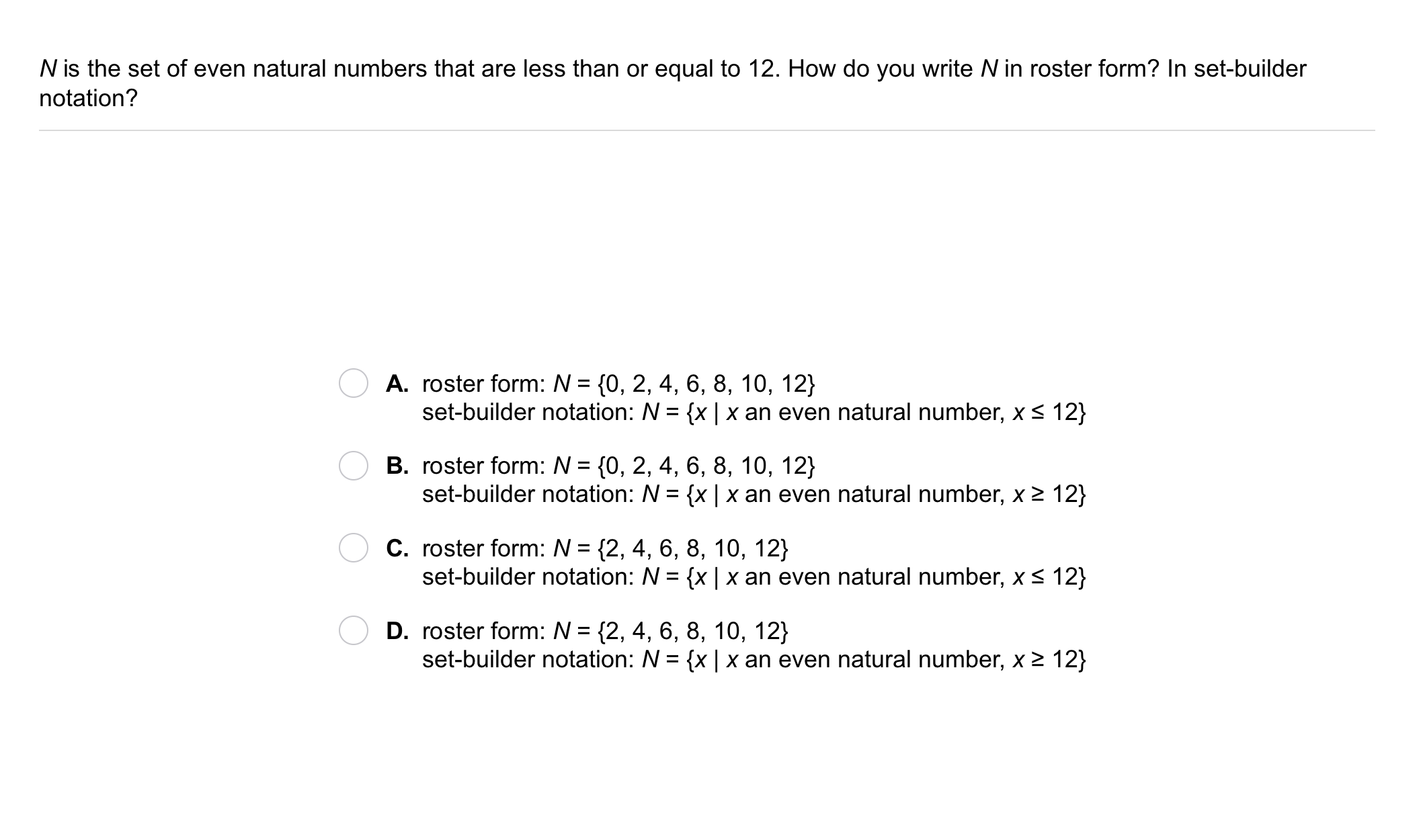2
2
10 pts
Problem 1 Got It?
A
B
C
D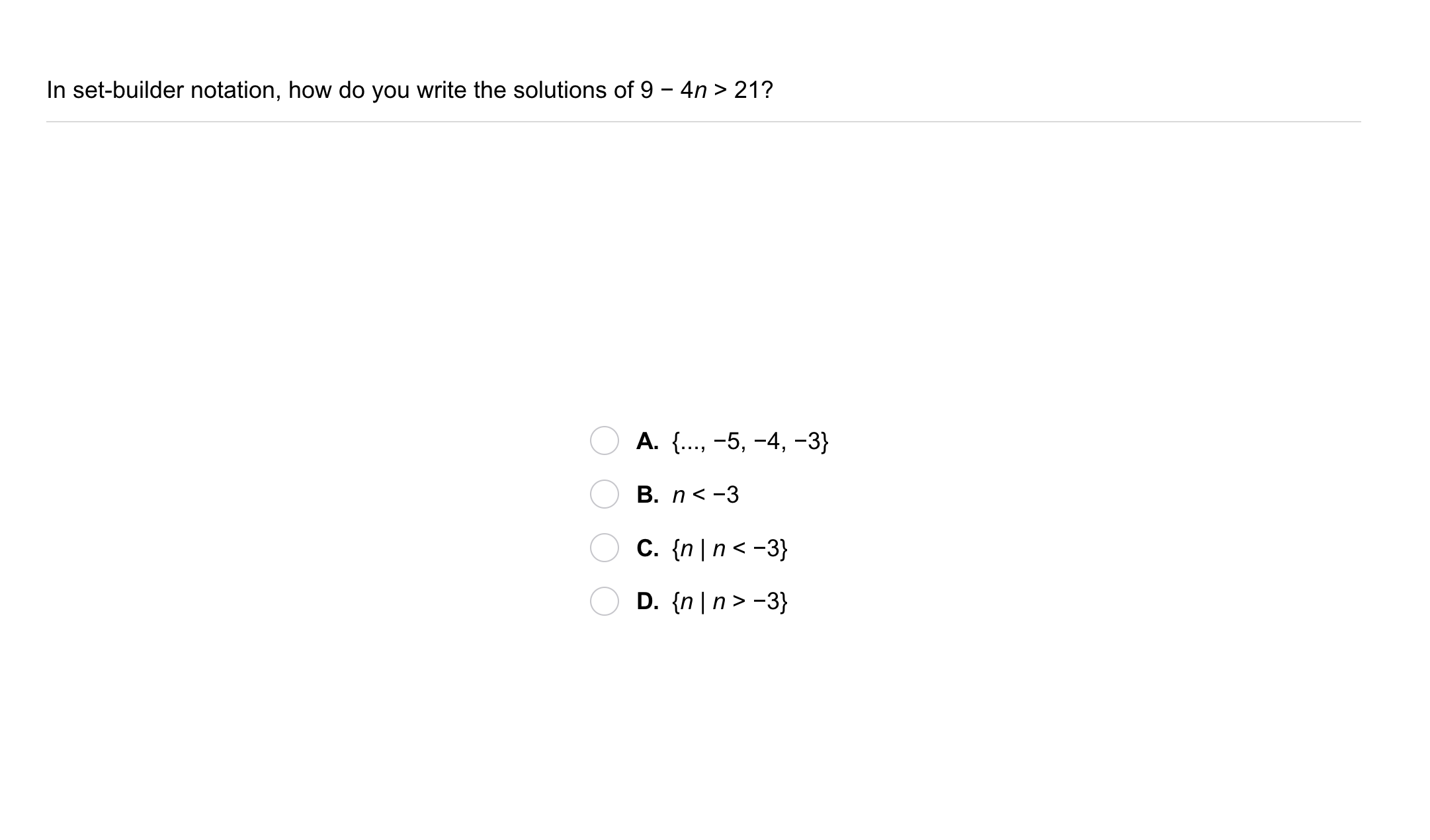3
3
10 pts
Problem 2 Got It?
A
B
C
D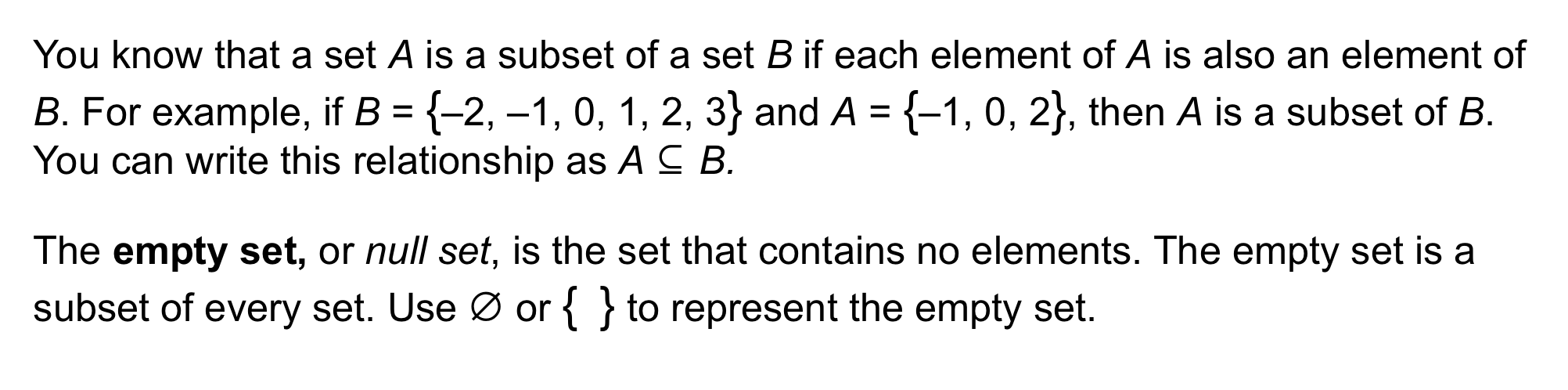4
10 pts
Problem 3 Got It? What are the subsets of the set P = {a, b}?
Select all that apply.
{a, b}
{a}
{} (aka ø or "the empty set")
{b}
5
10 pts
Problem 3 Got It? What are the subsets of the set S = {a, b, c}?
Select all that apply.
{a, b}
{d}
{a, b, c}
{c}
{b}
{a}
{a, c}
{} (aka ø or "the empty set")
{b, c}
6
5 pts
Problem 3 Got It? Reasoning: Let A = {x | x < -3} and B = {x | x ≤ 0}. Is A a subset of B?
Yes
No
7
10 pts
Problem 3 Got It? Reasoning: Explain your reasoning on the previous item.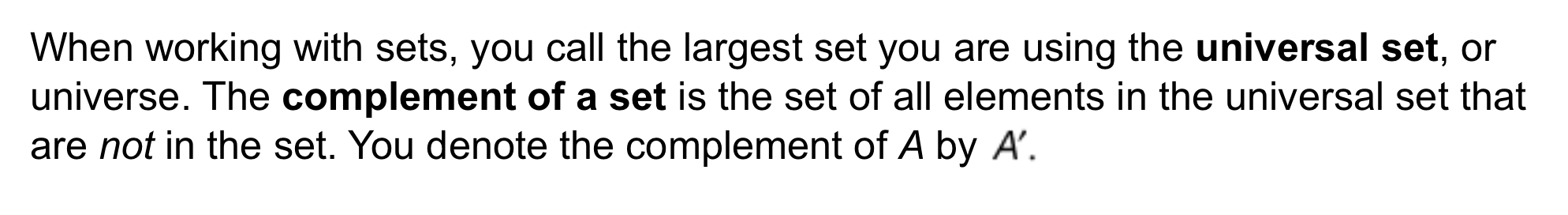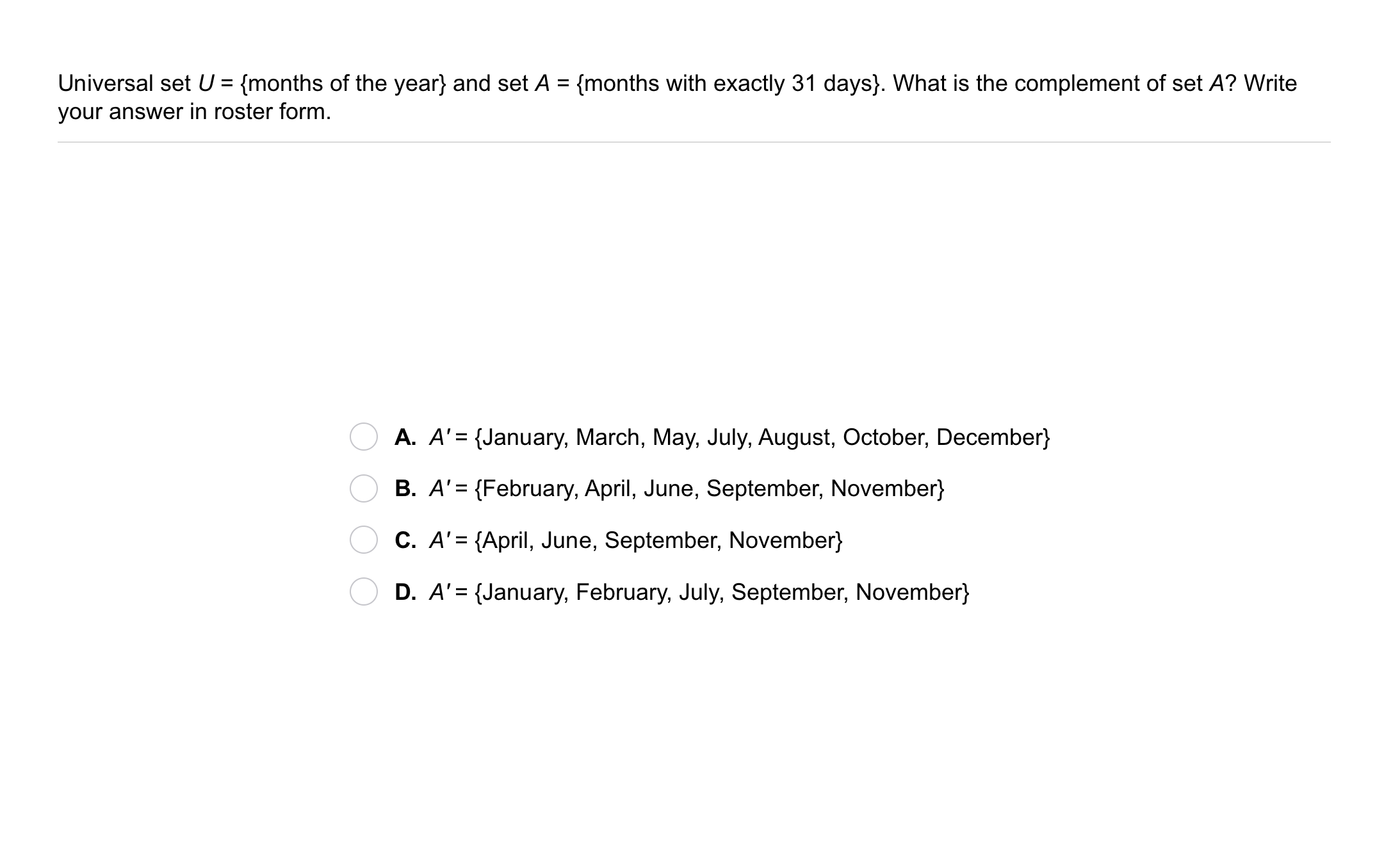8
8
10 pts
Problem 4 Got It?
A
B
C
D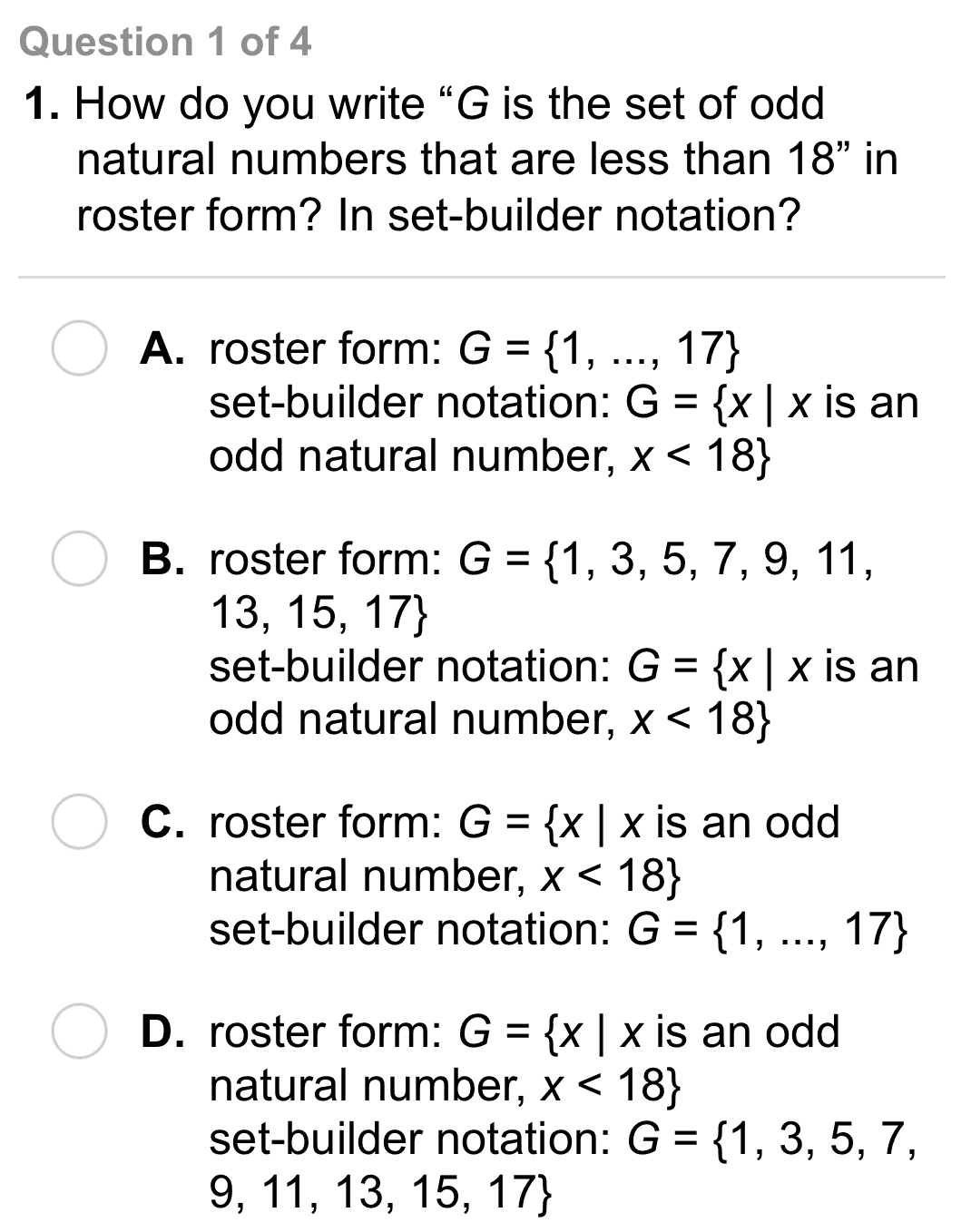9
9
10 pts
A
B
C
D10
10
10 pts
A
B
C
D11
11
10 pts
A
B
C
D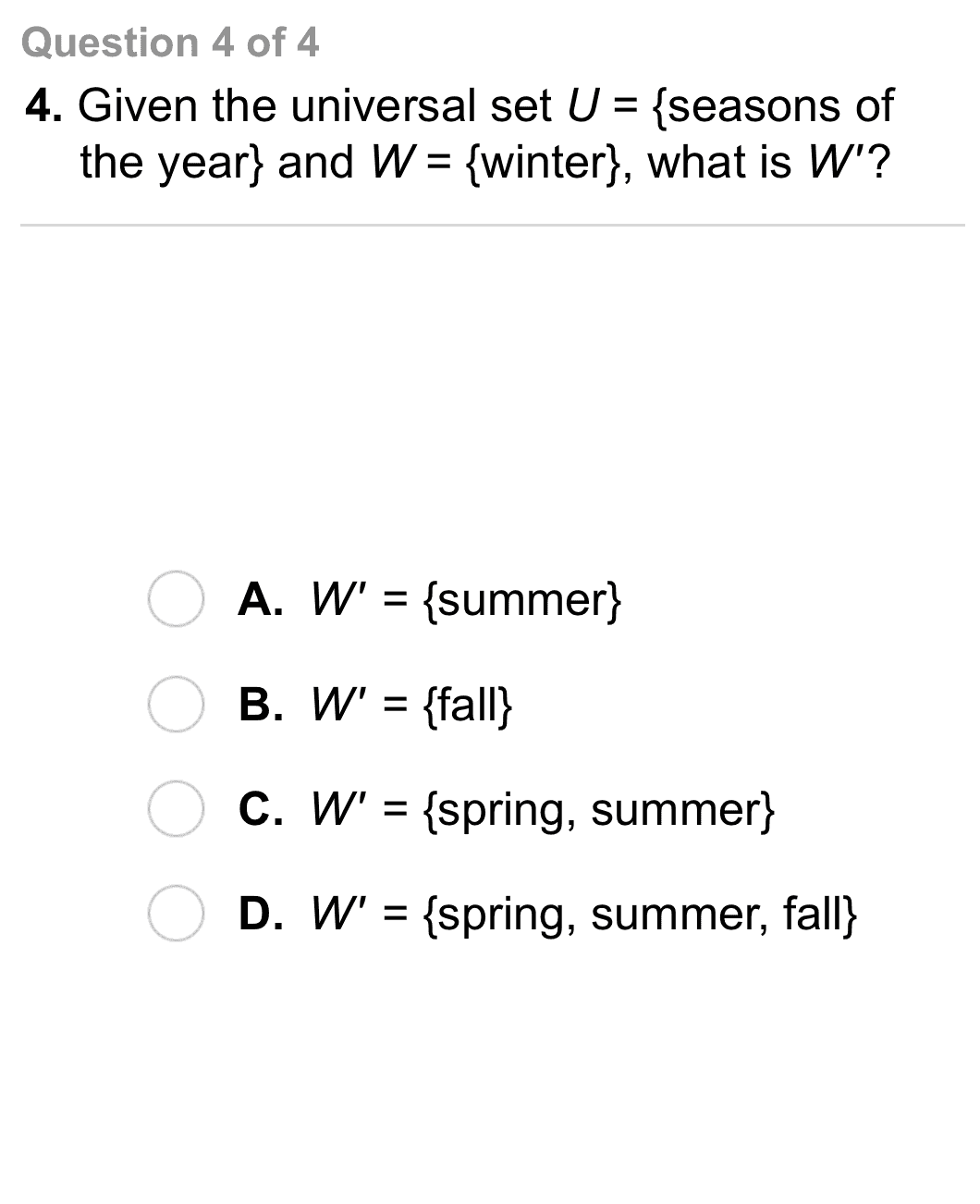12
12
10 pts
A
B
C
D13
10 pts
Vocabulary: What is the complement of a set A? Explain.
14
10 pts
Vocabulary: Categorize the subsets into the set(s) to which they belong. Some subsets may belong to multiple sets or not set at all.

Note that not all subsets for every set are included in this item.
• {}
• {3}
• {1, 3}
• {1, 3, 5}
• {1, 3, 5, 7}
• Set {1, 3, 5}
• Set {1, 3}
• Set {3}
15
10 pts
Reasoning: A nonempty set is a set that contains at least one element. Given nonempty sets A and B, suppose that AB. Is BA always, sometimes, or never true?
Always
Sometimes
Never
17
10 pts
Error Analysis: A student says sets A and B below are the same. What error did the student make?

A = {x| x is a whole number less than 5} B = {1, 2, 3, 4}18
5 pts
Review Lesson 3-4: Solve the inequality.

b < 6
b > 8
b < 8
b > 6
19
5 pts
Review Lesson 3-4: Solve the inequality.

t ≤ -5
t ≤ 5
t ≥ 5
t ≥ -5
20
5 pts
Review Lesson 3-4: Solve the inequality.

z > 2
z < 13
z < 2
z > 13
21
5 pts
Review Lesson 1-2: Evaluate the expression for the given value of the variable.
Enter only a number.
22
5 pts
Review Lesson 1-2: Evaluate the expression for the given value of the variable.
Enter only a number.
23
5 pts
Review Lesson 1-2: Evaluate the expression for the given value of the variable.
Enter only a number.
24
10 pts
Review Lesson 3-1: Graph the pair of inequalities on the same number line.25
10 pts
Vocabulary Review: Identify the numbers in the left column that are NOT whole numbers.

• NOT whole numbers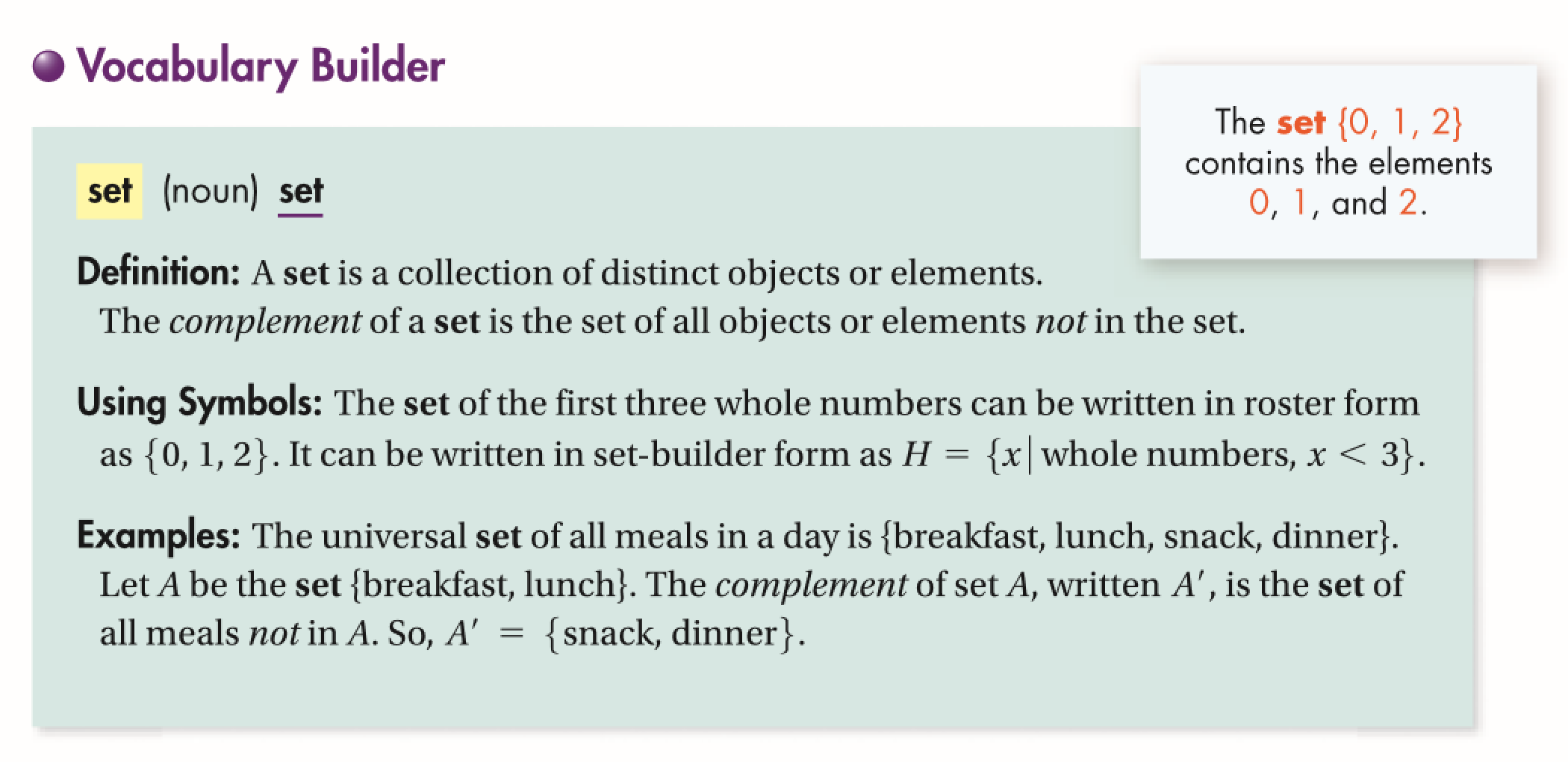26
10 pts
Use Your Vocabulary: Complete each set on the right with the most appropriate element from the left.
• sister
• cat
• mouth
• I
• {eyes, ears, nose, _?_}
• {mother, father, brother, _?_}
• {A, E, _?_, O, U}
27
10 pts
Use Your Vocabulary: Suppose that the universal set of coins is {penny, nickel, dime, quarter}. Let D be the set {penny, quarter}. What is the complement of set D ?

Drag and drop elements from the left to create the complement of set D on the right.
• penny
• nickel
• dime
• quarter
• Complement of D
28
100 pts
Notes: Take a clear picture or screenshot of your Cornell notes for this lesson. Upload it to the canvas. Zoom and pan as needed.